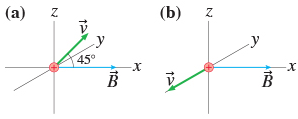# Problem: A proton moves in the magnetic field B? =0.40i^T with a speed of 1.0 x 107m/s in the directions shown in the figure.-In Figure (a), what is the magnetic force F on the proton? Give your answers in component form.F = ???    N-In Figure (b), what is the magnetic force F on the proton? Give your answers in component form.F = ???     N

###### FREE Expert Solution

Magnetic force:

$\overline{)\stackrel{\mathbf{⇀}}{\mathbf{F}}{\mathbf{=}}{\mathbf{q}}\stackrel{\mathbf{⇀}}{\mathbf{v}}\stackrel{\mathbf{⇀}}{\mathbf{B}}{\mathbf{s}}{\mathbf{i}}{\mathbf{n}}{\mathbf{\theta }}}$

(a)

q = 1.6 × 10-19 C

v = 1.0 × 107m/s

B = 0.40 T

θ = 45°

The field is directed in the positive x-direction. The velocity is directed in the positive z-direction making an angle of 45° with the field. Thus, the resultant force will be in the y-direction.

F = (1.6 × 10-19)(1.0 × 107)(0.40) sin 45 = 4.5 × 10-13 N j

83% (108 ratings)###### Problem DetailsA proton moves in the magnetic field B? =0.40i^T with a speed of 1.0 x 107m/s in the directions shown in the figure.

-In Figure (a), what is the magnetic force F on the proton? Give your answers in component form.

F = ???    N

-In Figure (b), what is the magnetic force on the proton? Give your answers in component form.

F = ???     N